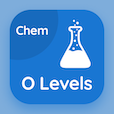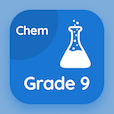Cambridge Online Courses (GCSE)

O Level Chemistry Quizzes

O Level Chemistry Quiz - Complete

Study Valency Table Multiple Choice Questions and Answers (MCQs), valency table quiz answers PDF, test 47 to download O Level Chemistry book. Chemical Formulae and Equations MCQ trivia questions, valency table Multiple Choice Questions (MCQ Quiz) for online college degrees. Valency Table Book PDF: measuring speed of reaction, pure substances and mixtures, chemical formulas, ph scale: acid and alkali, valency table test prep for GRE subject tests.

"Valency is numerically equal to the" Quiz PDF: valency table App APK with combining power of an atom, charge of an ion, combining power of a radical, and all of above choices for ACT test. Learn chemical formulae and equations questions and answers to improve problem solving skills for GRE prep classes.

## Valency Table MCQ Questions

MCQ: Valency is numerically equal to the

charge of an ion
combining power of an atom
all of above

MCQ: PH values have a range of

1 to 7
0 to 10
1 to 14
0 to 14

MCQ: The chemical formula of calcium nitrate is

CNOH
Ca(NO3)2
CaNO3
CaNO3

MCQ: What is true about pure substances?

they have fixed and exact boiling point
they have fixed and exact melting points
they form only one post on chromatogram
all of above

MCQ: During a chemical reaction, the

mass of catalyst remains unchanged
physical state of catalysts remain unchanged
changes in the chemical composition of catalysts may be observed
chemical composition of the catalyst may change

### More Quizzes from O Level Chemistry Book